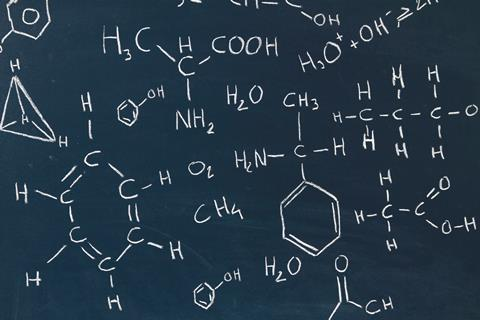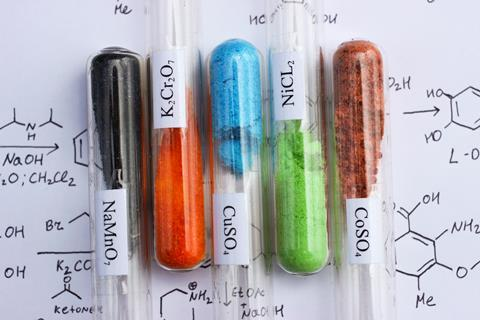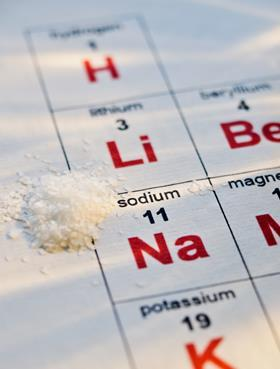Ollie Hunter suggests ideas, resources and activities for your classroomHave you ever put Epsom salts in your bath, taken Milk of Magnesia for indigestion or put flowers of sulfur on your roses? You probably know the chemical compositions of these products, but what about spirit of hartshorn or oil of vitriol; and could you make Glauber’s salt?

These alchemic names may be evocative and mysterious but they don’t communicate chemical knowledge well. Although we still cling on to a few of these names, most chemicals are now named systematically on the basis of their composition. Despite this, to a novice chemist, ammonia and sulfuric acid can be equally as ambiguous as spirit of hartshorn or oil of vitriol. Even systematic names like copper chloride only provide partial information – the quantitative relationship between the elements is missing.

Similar problems exist when describing reactions. Natural language is very good at naming things, but has difficulty with expressing exact amounts ‒ that’s why we use numbers. Imagine trying to answer the question: ‘How old are you?’ without the use of numbers.

Download a lesson activity on writing formulas for non-metal compounds [MS PowerPoint or pdf], and a student worksheet [MS Word or pdf].

Two hundred years ago Swedish chemist Jöns Jacob Berzelius introduced a symbolic system for describing the chemical make-up of substances. Symbols, usually the first or first two characters of the element’s name, combined with numbers to show the relative amounts present. The elements in these formulas where joined with a +. So, copper oxide became Cu+O and water became 2H+O. These formulas removed both the mystery of composition caused by the alchemic name and the lack of quantitative information. Berzelius’s system developed over the years into the chemical formulas we recognise today: CuO and H2O.### What students need to know

Chemical equations and formulas are a vital part of learning chemistry from 11‒19. Being able to use them correctly is part of what it means to be a chemist. Formulas and equations allow chemists to communicate chemical knowledge efficiently.

Students should understand that chemical formulas:

• provide a name for the substance – eg K2SO4 is potassium sulfate;
• provide a list of the elements within the substance – eg potassium, sulfur and oxygen;
• provide a ratio of the elements in the substance – eg two parts potassium, 1 part sulfur, 4 parts oxygen;

and that chemical equations:

• describe how substances change ‒ eg carbon + oxygen → carbon dioxide becomes C + O2 → CO2;
• tell us the ratio of reactants and products in a reaction ‒ eg 2Mg + O2 → 2MgO means two lots of magnesium reacts with one lot of oxygen to give two lots of magnesium oxide;
• can include state symbols ‒ (g), (l), (s) and (aq) ‒ that can help students visualise a reaction, and explain observations.

Students should be able to:

• write formulas of elements and simple compounds using chemical symbols and numbers.
• name simple compounds from a chemical formula using prefixes such as mono-, di-, tri- and words such as oxide, chloride, hydroxide, sulfate, nitrate and carbonate.
• construct word equations for reactions.
• represent the reactants and products of a chemical reaction in a balanced equation.

A general teaching sequence for formulas and equations could be:

• carry out simple test-tube or microscale reactions;
• learn the general word equations for the chemical reactions;
• learn to write specific word equations;
• learn how to construct chemical formulas;
• and use chemical formulas and the word equation to construct balanced chemical equations.Chemical names are mostly systematic, but students need to know that it isn’t always so; maybe take a pinch of salt with that name?

Two excellent resources for learning how to write word equations can be found on Learn Chemistry.

Once students are confident with word equations, you can turn to writing chemical formulas. Unfortunately the names of some substances don’t always translate directly into chemical formulas. For example, the formulas of water, methane, ammonia, nitric acid etc will have to learnt by heart. Display the names and formulas and give students strategies to commit these to memory.

Naming non-metallic compounds starts with understanding the prefixes mono-, di-, tri- etc. Link these with words from everyday life, and see how many the students can come up with. Then break down the formulas of some compounds they may have already heard of. For example, carbon dioxide as carbon with two oxygens. Plenty of examples will be needed to help students develop a mastery of these conversions. You can then ask the students to make up their own examples, and challenge their peers to do the conversions.

Finally, you can move onto naming salts and ionic compounds. Again, some rote learning will be required to ensure they know names of polyatomic ions such as ammonium, hydroxide and carbonate.

The RSC Assessment of Learning resource will prove useful here.

### Common misconceptions

• All formulas as representing molecules, eg ‘NaCl means sodium chloride molecules.’
• Not recognising polyatomic ions as a distinct chemical species, eg MgSO4 named as magnesium sulfur tetroxide rather than magnesium sulfate.
• Confusing polyatomic ions as elements, eg ’I can’t find hydroxide on the periodic table.’
• Mixing up the symbol for an element, eg Cl, and its formula as a substance, eg Cl2. This can be complicated by the use of the element symbol for giant structures – eg diamond as C and iron Fe – and some seemingly illogical exceptions, eg S8 and P4.
• Mixing up the formula of a substance with the chemical equation of its production. For example giving ‘hydrogen + oxygen → water’ when asked for the formula of water. This misconception stems from maths where the two words are often used interchangeably and everyday life where the word formula is often associated with how something is made.
• Students often struggle with the numerical relationships in equations and formulas, this can make the balancing of equation and chemical calculations difficult.

### Formative assessment

Writing formulas and equations is not a skill that will develop after one or two lessons. It is a skill that should be an ongoing part of chemistry lessons. Use regular low stakes testing of word equations and simple chemical equations alongside the recall of formulas. Focus on the diatomic elements, as well as common acids, bases and salts to help build students overall chemical knowledge and confidence. Games of formula or equation bingo are popular, and mini whiteboards are useful for quick formative assessment.

### Take-home points

• Formulas are not just shorthand for the chemical name but provide information about the relative amounts of the elements in a substance.
• The formulas of some compounds cannot be derived from the name of the substance and will need to be learnt.
• Writing chemical formulas and equations needs frequent reinforcement.
• Don’t rush into writing balanced equations. Help students master writing formulas and word equations first.

### Progression to 14‒16

Once students have mastered writing formulas and balanced chemical equations, these can be extended to include writing ionic equations for precipitation reactions and half equations for electrochemical and redox processes.

At this level, formulas and equations are used to perform many chemical calculations including relative formula mass, moles of substance, reacting mass and titration calculations. Mastering these formulas and equations helps smooth progression through these calculations.

Ollie Hunter is head of chemistry and EPQ Coordinator at Benenden School, Kent and a part-time EdD student at the Faculty of Education University of Cambridge

### What is hidden in formulas and equations?

Balancing equations and completing chemical calculations require clear understanding of the role of numbers in formulas. The difference between numbers in front of and numbers within formula can cause confusion.

A number within a formula means multiples of the element (or groups of elements) to the left of the number.

So the formula for copper hydroxide Cu(OH)2 is read as:

1 x Cu + 2 x (O+H) or Cu + 2 x O + 2 x H

A more complex formula, such as ammonium sulfate (NH4)2SO4, is read as:

2 x (N + (4 x H)) + 1 x S + 4 x O or 2 x N + 8 x H + S + 4 x O

A number used in front of a formula in an equation tells us how many molecules (or moles) of the substance react. For example, in the equation 2H2 +O2 →  2H2O is read as:

2 x H2 + 1 x O2 → 2 x (H2O)

or

two molecules of hydrogen react with one molecule of oxygen to give two molecules of water.

Be aware that some students assume the number in front of chemical formula is part of the formula, or only refers to the first element. For example interpreting 2NaOH as:

’2NaOH is the formula of sodium hydroxide’ or 2 x Na + O + H